Latest Banking jobs   »

# Quantitative Aptitude Quiz For FCI Phase I 2022- 8th December

Directions (1-10): In each of these questions, two equations (I) and (II) are given. You have to solve both the equations and give answer
(a) if x>y
(b) if x≥y
(c) if x<y
(d) if x ≤y
(e) if x = y or no relation can be established between x and y.

Q1. I. x2 – 1 = 0
II. y2 – y – 2 = 0

Q2. I. x2 + x – 12 = 0
II. y2 – 8y + 15 = 0

Q3. I. 24×2 – 59x + 36 = 0
II. 20y2 + 23y + 6 = 0

Q4. I. 20×2 – 17x + 3 = 0
II. 56y2 + 2y – 4 = 0

Q5. I. 28×2 + x – 15 = 0
II. 21y2 – 17y – 30 = 0

Q6. I. 6x² + 7x + 2 = 0
II. 3y² + 8y + 5 = 0

Q7. I. 7x² – 23x + 6 = 0
II. y² – 7y + 12 = 0

Q8. I. 5x² + 13x – 6 = 0
II. 2y² + 13y – 7 = 0

Q9. I. 4x + 3y = 4
II. 6x + 5y = 8

Q10. I. x² – 19x + 88 = 0
II. y² + y – 56 = 0

Directions (11 – 15): In each of these questions, two equations (I) and (II) are given. You have to solve both the equations and answer the following questions.
(a) x > y
(b) x < y
(c) x ≥ y
(d) x ≤ y
(e) x = y or no relation

Q11. I. 2x² – 11x + 15 = 0
II. 2y² – 13y + 20 = 0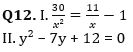Q13. I. x² – 84 = 112
II. y³ + 1845 = 5220

Q14. I. x² + 18x + 80 = 0
II. y² + 14y + 48 = 0

Q15. I. 18x – 12y = 9
II. 16y – 20x = 2

Solutions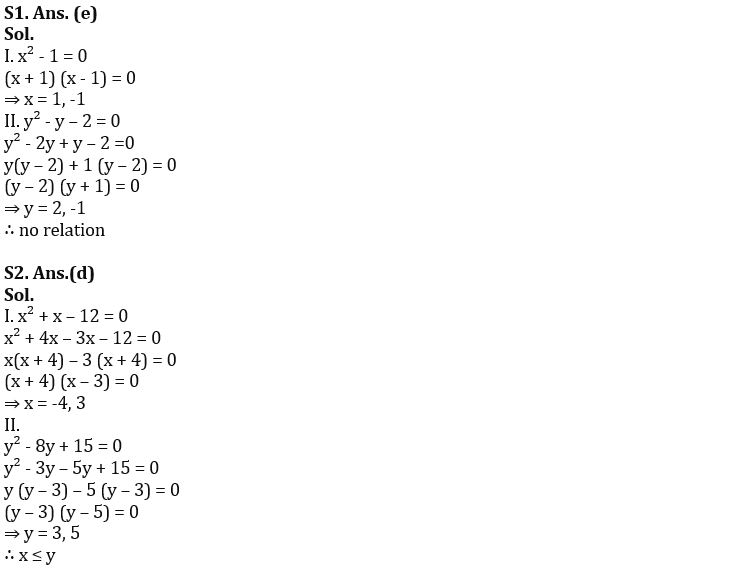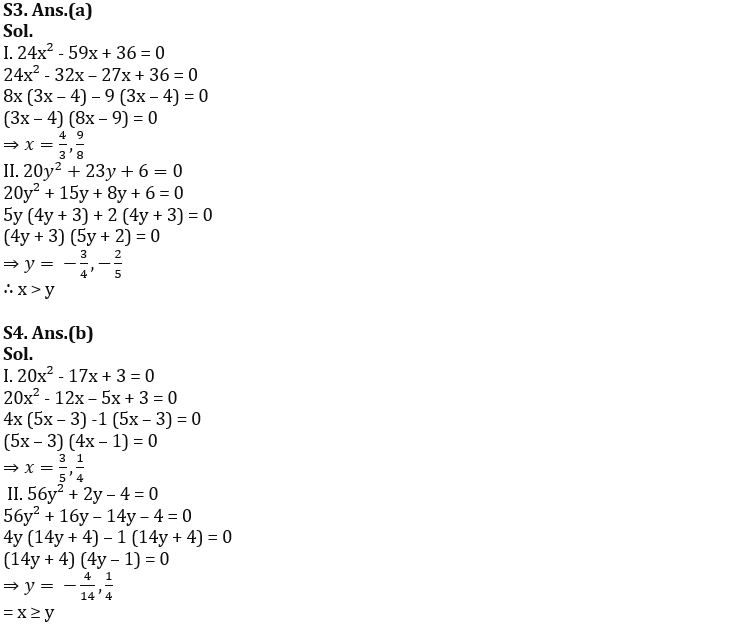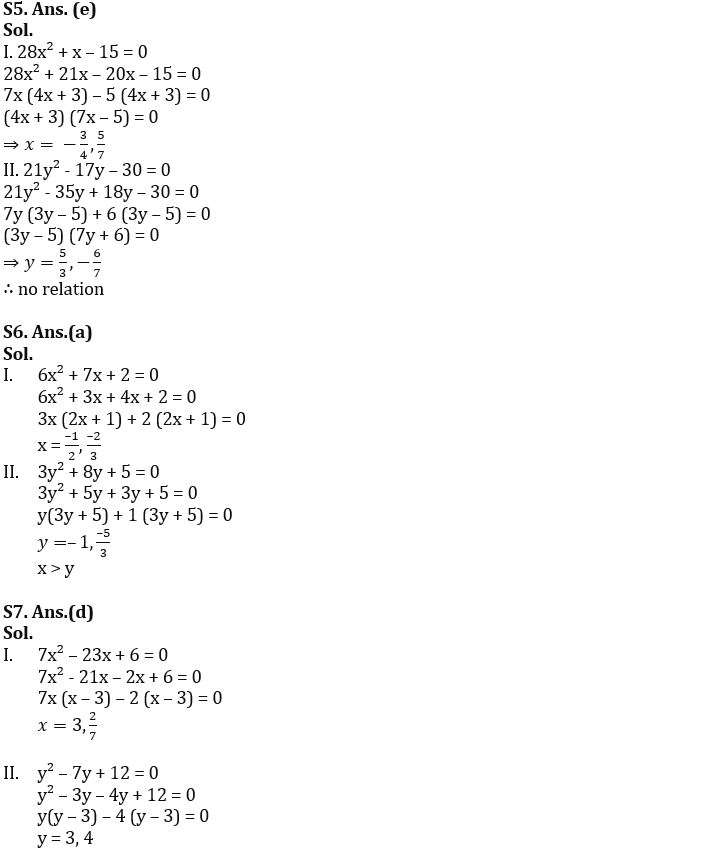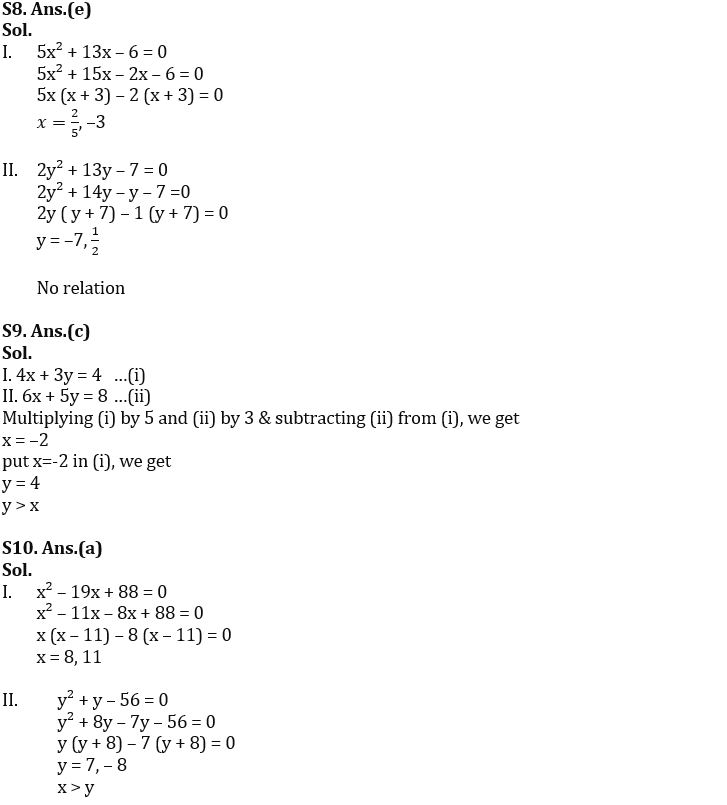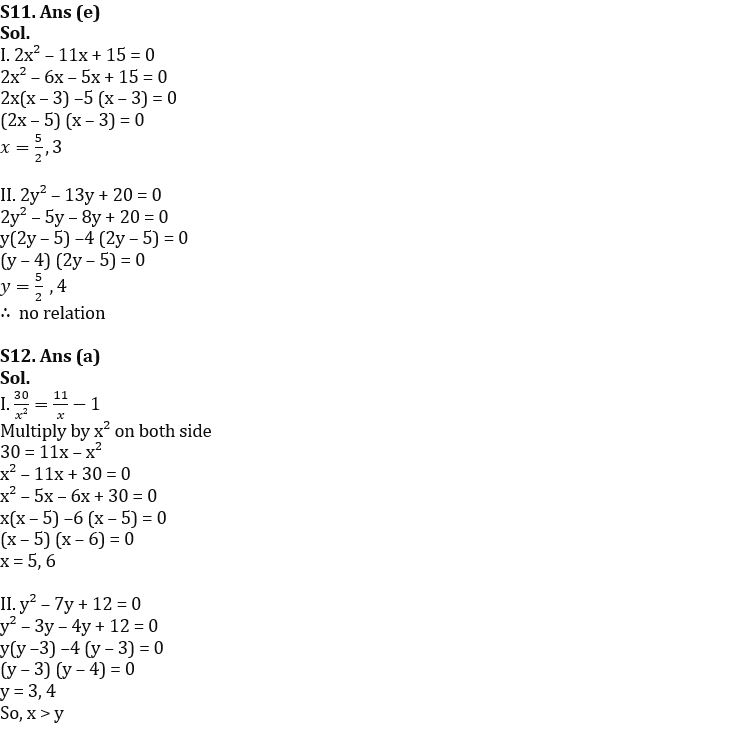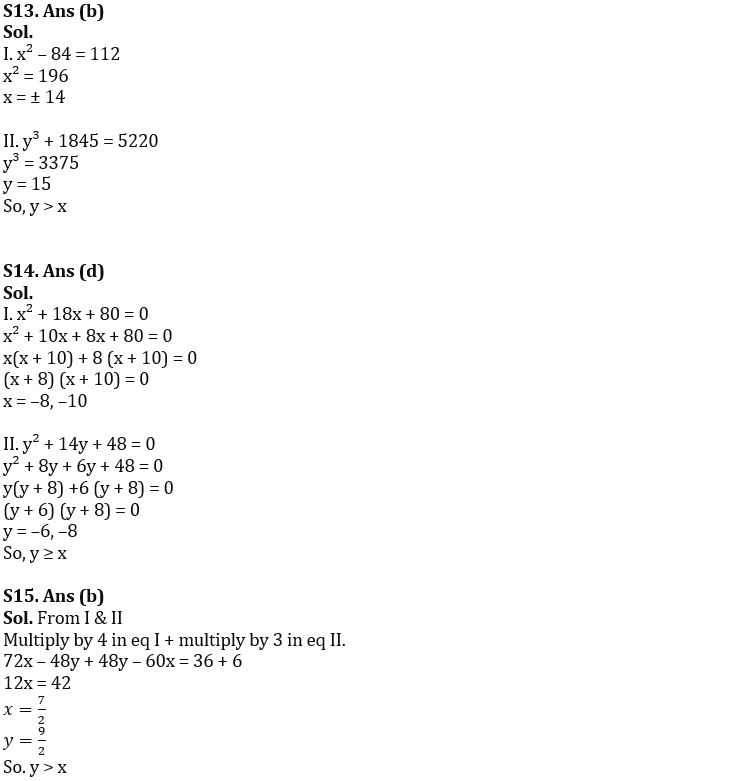#### Congratulations!Union Budget 2023-24: Free PDF